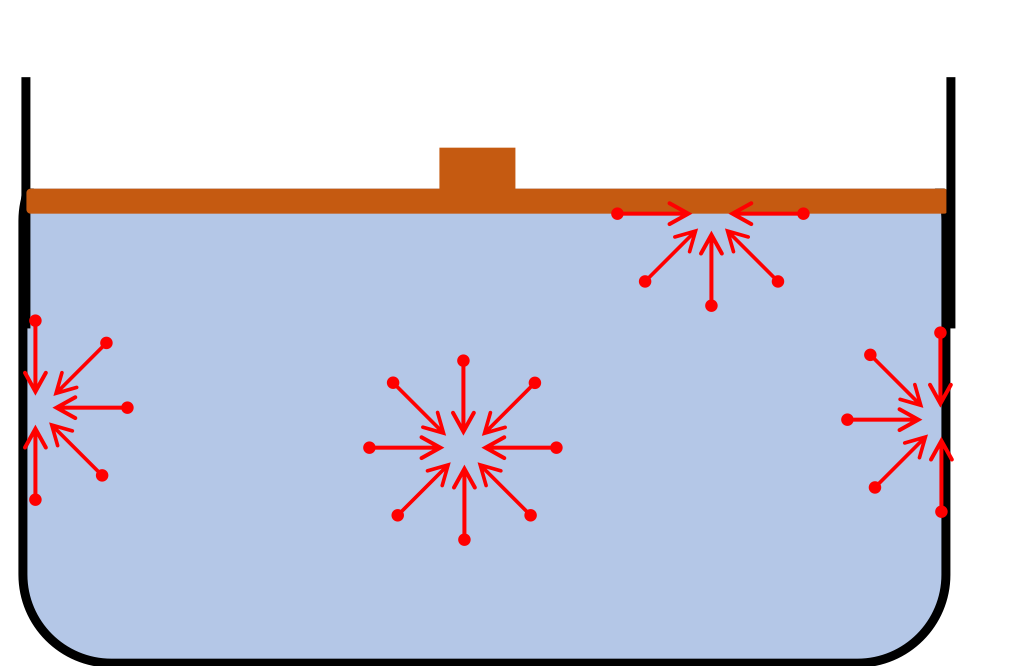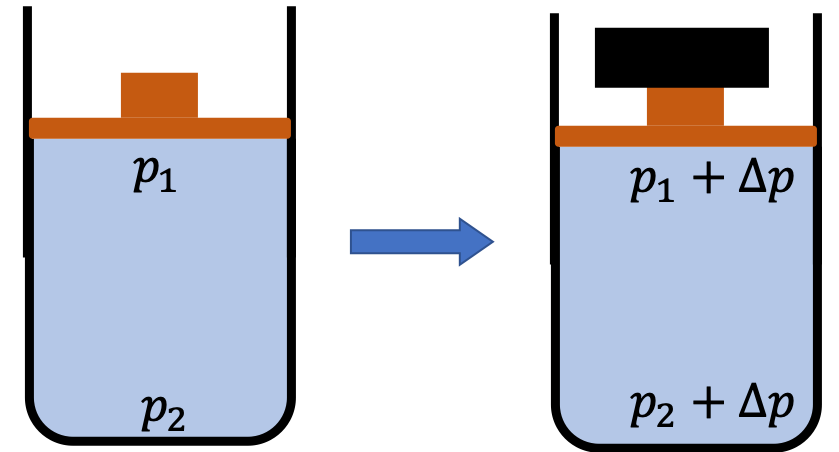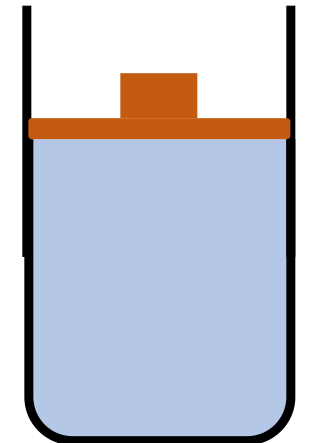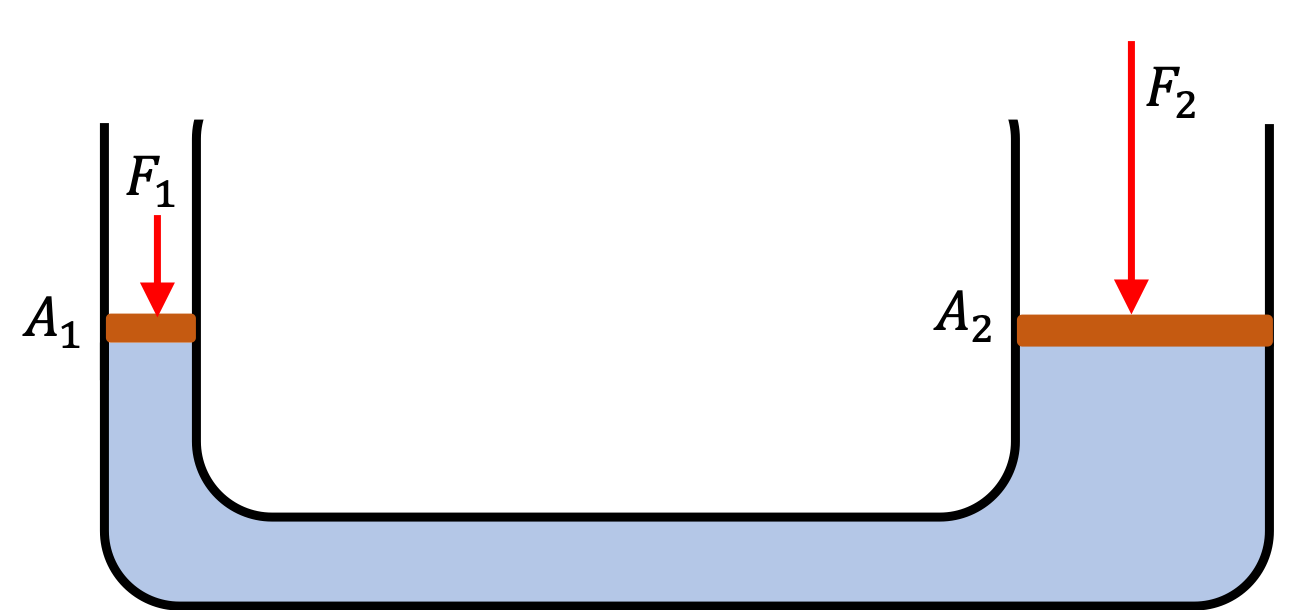## Section17.5Pascal's Principles

When a fluid is not flowing, we say that the fluid is in a static equilibrium. In this condition, the net force on any part of a fluid at rest must be zero, otherwise the fluid will start to flow.

In 1653 the French mathematician, and physicist Blaise Pascal (1623-1662) published his Treatise on the Equilibrium of Liquids in which he outlined three principles of static fluids.

### Subsection17.5.1Pascal's First Principle

According to this principle:

The force at any point inside a fluid due to the pressure at that point acts with the same magnitude in all direction.

The direction is important here. As a consequence of the direction, the force on the walls of the container due to the pressure of the fluid is perpendicular to the wall.### Subsection17.5.2Pascal's Second Principle

According to this principle:

When pressure is changed (increased or decreased) at any point in an incompressible fluid, the pressure at all other points in the fluid changes to the same extent.

The key phrase here is change in pressure. Often, the second principle of Pascal is also referred to as the Pascal's principle. Note that this principle does not say that the pressure is same at all points of a fluid, which is certainly not correct, since we already know that the pressure in a fluid varies with depth. This principle rather addresses the change in pressure.

Figure 17.5.1 illustrates Pascal's principle of same change in pressure everywhere.Figure 17.5.1. Pressure in fluid is changed when the fluid is pressed by placing additional weight on the moveable lid. Although, the pressure at the top layer of the fluid is different than at the bottom layer, they both increase by the same amount $\Delta p \text{.}$

A freely movable piston of mass 100 kg covers water in a rigid cylinder of radius 5 cm touching the surface of the water.

(a) What is the pressure at a depth of 50 cm from the top of water?

(b) A block of steel of mass 300 kg is placed on the piston. What is the new pressure at a depth of 50 cm from the top?Hint

(a) $p_0 + mg/A + \rho g h \text{,}$ (b) $mg/A$ and Pascal's second principle.

(a) $2.31\times 10^5\text{ Pa}$ , (b) $6.05\times 10^5\text{ Pa}\text{.}$

Solution 1 (a)

(a) The pressure at $50\text{ cm}$ depth will be the sum of atmospheric pressure, the pressure due to the weight of the piston, and the $50\text{-cm}$ column of water above the point where we want the pressure. We need area of cross-section.

\begin{equation*} A = \pi R^2 = \pi \times 0.05^2 = 0.007854\text{ m}^2. \end{equation*}

Therefore,

\begin{align*} p \amp = p_0 + \dfrac{mg}{A} + \rho g h, \\ \amp = 1.013\times 10^{5} + \dfrac{100\times 9.81}{0.007854} + 1000\times 9.81\times 0.50,\\ \amp = 2.31\times 10^5\text{ Pa}. \end{align*}
Solution 2 (b)

(b) According to Pascal's second principle, the new pressure will be the old pressure plus the additional pressure from new weight.

\begin{equation*} 2.31\times 10^5 + \dfrac{300\times 9.81}{0.007854} = 6.05\times 10^5\text{ Pa}. \end{equation*}

A cylindrical tank containing oil is under pressure such that the pressure at the bottom is $3.0\text{ atm}\text{,}$ while the pressure at the top is $1.5\text{ atm}\text{.}$ The cover of the container is removed so that the pressure at the top is now $1.0\text{ atm}\text{.}$ What is the pressure at the bottom now?

Hint

Change in pressure as governed by Pascal's second principle.

$2.5\text{ atm}$

Solution

Since the pressure at the top decreased by $0.5\text{ atm}\text{,}$ the same should happen at all points in the tank. Therefore, the pressure at the bottom will now be $3.0-0.5 = 2.5\text{ atm}\text{.}$

### Subsection17.5.3Pascal's Third Principle

Pascal's third principle addresses the operation of a hydraulic jack.

In a hydraulic jack, a small force applied over a small area can balance a much larger force on the other side over a larger area.

A hydraulic jack is a device that contains an incompressible fluid in a U-tube fitted with moveable pistons on the two sides. One side of the U-tube is narrower than the other as shown in Figure 17.5.4. We understand the reason behind the working of the hydraulic jack as follows.Figure 17.5.4. A hydraulic jack. A smaller force acting on a smaller area can produce as much pressure as a larger force acting on a larger area. The ratio $F_2/F_1 = A_2/A_1$ is the mechanical advantage of the hydraulic jack.

When a force $F_1$ is applied on the side with the area of cross-section $A_1$ it adds pressure $P_1$ to all points of the fluid according to the second principle stated above.

\begin{equation*} P_1 = \dfrac{F_1}{A_1} \end{equation*}

This pressure when transmitted through the internal stress in the fluid acts on the other piston in the upward direction. Therefore, a second force $F_2$ must be applied on the piston $A_2$ to balance the effect of the increased pressure $P_1\text{,}$ which provides pressure $P_2 = F_2/A_2\text{.}$ Balancing of the two increases in pressure from two opposite directions is the statement of the third principle.

\begin{equation} \dfrac{F_1}{A_1} = \dfrac{F_2}{A_2}.\tag{17.5.1} \end{equation}

You can rearrange this equation to show the force multiplication occuring in a hyhraulic jack.

\begin{equation} \dfrac{F_2}{F_1} = \dfrac{A_2}{A_1}.\tag{17.5.2} \end{equation}

If $A_2>A_1\text{,}$ a smaller force $F_1$ can support a larger force $F_2\text{.}$ That is why the ratio $\dfrac{F_2}{F_1}$ is called the mechanical advantage of a hydraulic jack.

Refer to Figure 17.5.6. (a) Find the force needed to balance a $3000\text{-kg}$ car on the wider side of a hydraulic jack with the circular cross-sections of radii $10\text{ cm}$ and $0.5\text{ cm}\text{.}$

(b) What is the mechanical advantage of this hydraulic jack?

Hint

Use Pascal's thrid principle.

(a)$73.6\text{ N}\text{,}$ (b) $400 \text{.}$

Solution 1 (a)

(a) From Pascal's third principle, it is immediately evident that we need the following force to balance the weight of the car placed on the wider side.

\begin{align*} F_1 \amp = \dfrac{A_1}{A_2}F_2 = \left( \dfrac{\pi r_1^2}{\pi r_2^2}\right)F_2 = \left( \dfrac{ r_1 }{ r_2 }\right)^2\,F_2. \end{align*}

With $F_2 = mg = 3000 \times 9.81 = 29430\text{ N}\text{,}$ we get

\begin{equation*} F_1 = \left( \dfrac{0.5}{10} \right)^2 \times 29430 = 73.6\text{ N}, \end{equation*}

which is the weight of a $7.5\text{-kg}$ block.

Solution 2 (b)

The mechanical advantage is the ratio of forces on the two sides of the hydraulic jack.

\begin{equation*} \dfrac{F_2}{F_1} = \dfrac{29430\text{ N}}{73.6\text{ N}} = 400. \end{equation*}

In a hydraulic jack, a $4000\text{-kg}$ pickup truck is supported on the side with cross-sectional area $0.3\text{ m}^2$ by a $100\text{-N}$ force act on the narrower end.

(a) Find the area of cross-section of the narrower end.

(b) A refrigerator weighing $3000\text{ kg}$ is loaded on the truck. Now, what force must be used to support the truck?

Hint

(a) Use hydraulic jack equation, (b) You do not need to start over, instead use the proportionality of the hydraulic jack.

(a) $7.65\text{ cm}^2\text{,}$ (b) $175\text{ N}\text{.}$

Solution 1 (a)

(a) We use the Hydraulic Jack equation

\begin{equation*} \dfrac{F_1}{A_1} = \dfrac{F_2}{A_2}, \end{equation*}

to find the area

\begin{align*} A_1 \amp = \frac{F_1}{F_2} A_2 \\ \amp = \dfrac{100}{4000 \times 9.81 }\ \times 0.3 = 7.65\text{ cm}^2. \end{align*}
Solution 2 (b)

(b) The areas are same but the weight on the truck side went from $4000\times g$ to $7000\times g\text{.}$ Therefore, the support force must go from $100\text{ N}$ to $(7/4) \times 100 = 175\text{ N}\text{.}$